Tutorial math and reading software for elementary and secondary arithmetic, basic math, algebra, geometry, precalculus plus GED, ABE, and CLEP preparation for elementary school, high school, college, adult education, and homeschool students.

# Arithmetic SeriesProgram 3: SUBTRACTION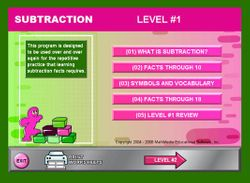Item# Subtraction
Regular price: \$49.00
Limited Time Offer: \$24.50
Media type::

## Product Description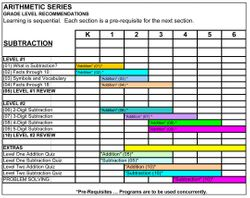[click on image]

Students learn subtraction from a variety of perspectives - counting, eliminating, graphing followed by practice, practice, practice. Once the basic subtraction concepts are learned, this math software program moves on 2-3-4-and-5-digit subtraction with borrowing - each step of the process is presented and practiced. Every screen is scored for immediate response to the student. Every four sections concludes with a comprehensive review. Extras include printable practice drills in addition and subtraction. Worksheets are printable for additional practice and reinforcement -- use as classwork or homework. Problem solving worksheets help the students learn to critically discern the differences between addition and subtraction. Worksheets include critical thinking discussions and practice about temperature, vocabulary (horizontal, vertical), figural analysis and analogies, mazes, morse code, classification practice, comparison of 2-dimensional and 3-dimensional figures, and reading coins (money).
These arithmetic topics are presented to classroom students over the course of years. Use this tutorial software to keep up with schoolwork, to learn at home, to remediate, to accelerate, as a course or as a reference.

## Accessories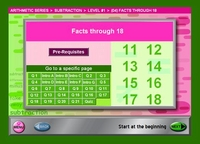Each section begins with a navigation page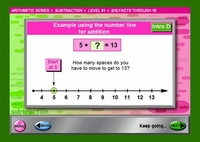Introductions unfold...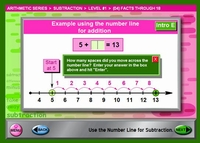Step-by-Step Introductions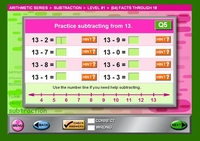Practice with HINTs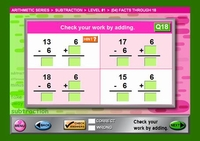More practice...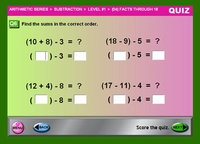Conclude each section with a Quiz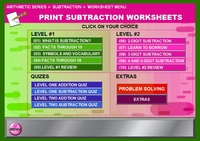Worksheets